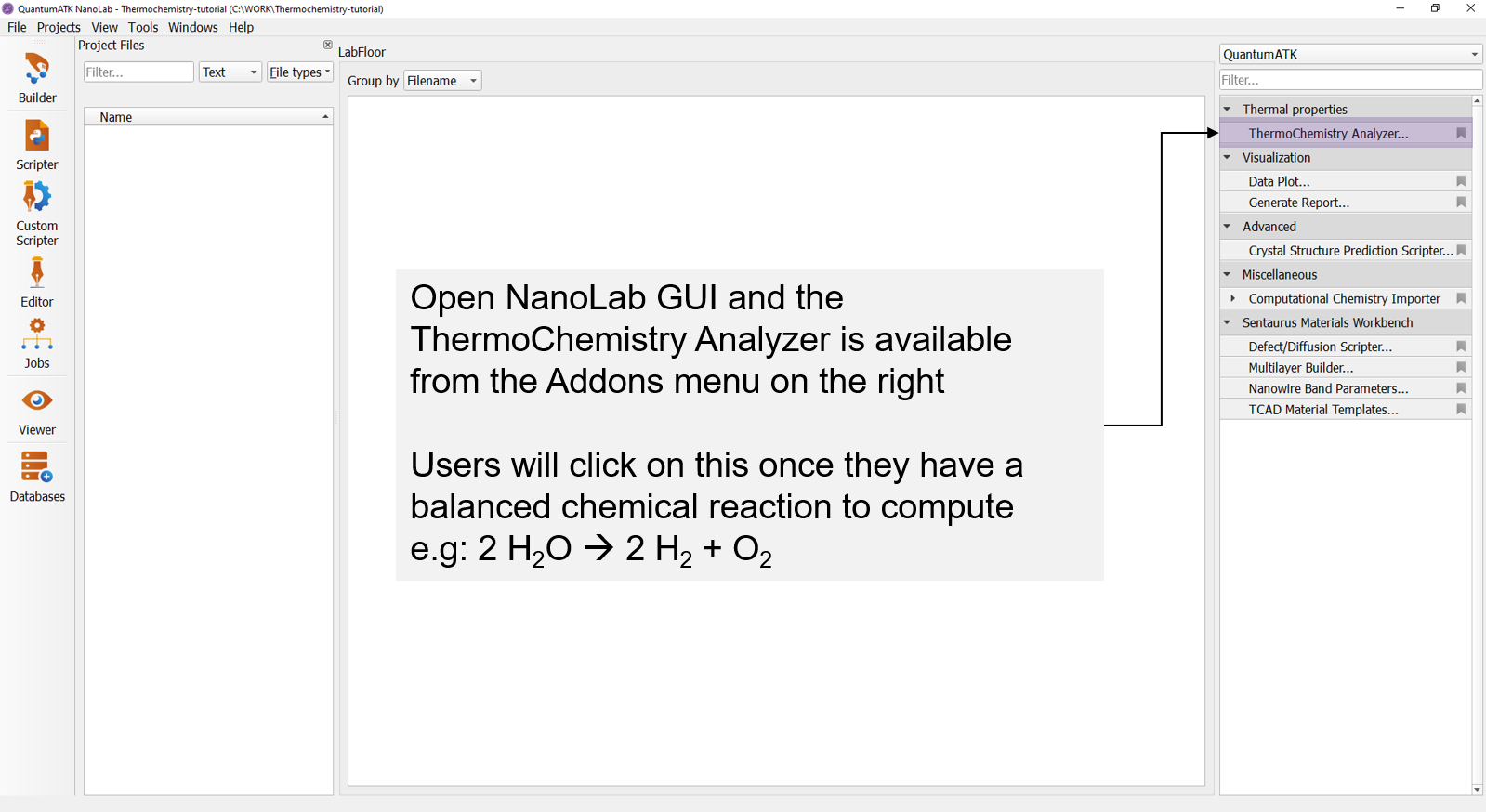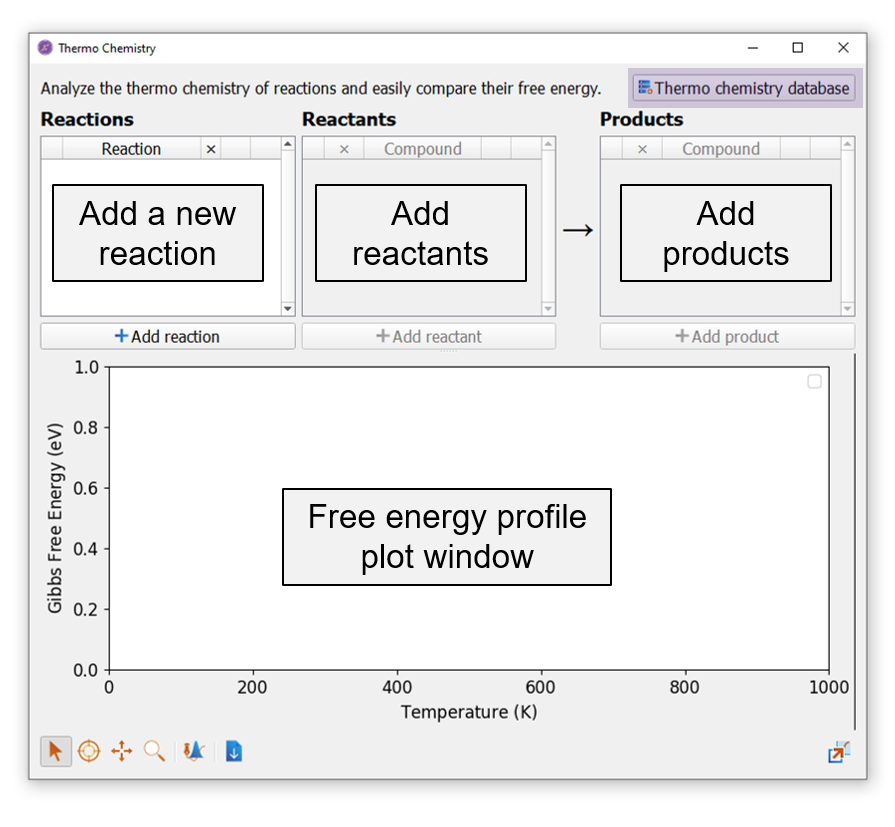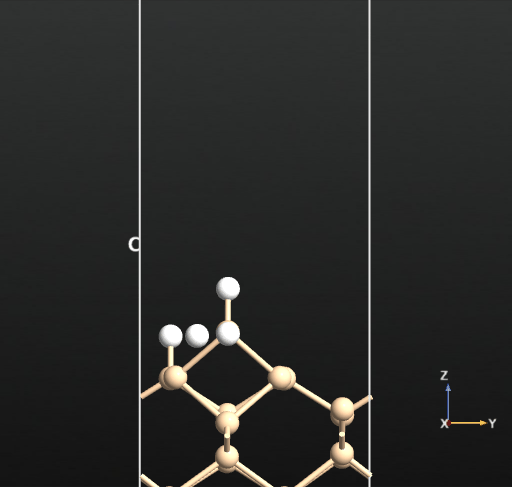# Simulating Si Deposition using Silane¶

Version: R-2020.09-SP1

In this tutorial, we will look at a QuantumATK workflow to simulate the reaction mechanisms involved in the deposition of Si using silane. We will perform surface-science style slab calculations using plane-wave DFT to study the adsorption and dissociation of silane along with the formation and desorption of H2 from the surface.## Background¶

Using thermal chemical vapor deposition (TCVD) technique, Si is deposited by gas phase pyrolysis of SiH4 . SiH4 molecules impinge on the Si substrate and donates H atoms to the Si surface followed by the deposition of SiHx . The H atoms on the surface form H2 molecules and desorb at elevated temperatures. This is the process we will simulate in this tutorial following the reference article [LRHL16]. This can be done in 3 steps. Step 1 involves computing the reference bare Si(100) surface in a supercell and an SiH4 molecule in the gas phase. In step 2, we study the adsorption and dissociation of SiH4 on the relaxed Si(100) surface. Step 3 concerns the formation and desorption of an H2 molecule from the Si surface.

## Getting started¶

Open the QuantumATK GUI, NanoLab, and select ‘Create New…’ in the Project Dialog. Create a new project in an empty folder with an appropriate name.

## Step 1: Reference Calculations¶

We will perform calculations for the reference SiH4 molecule and the bare Si(100) surface using a similar setup as the reference article [LRHL16]. The relevant python scripts are found here SiH4.py, Si-alpha-100.py. Panel a in the figure below shows the SiH4 molecule in a simulation box. Panel b in the figure shows the side and top views of the reconstructed Si(100) surface. From the top view one can see the Si-dimer formation on the surface and these are the reactive sites for SiH4 adsorption and dissociation. Total electronic energies of these two calculations are summed up to give the reference energy value of the noninteracting system for adsorption energy calculations. The adsorption energy is the difference between the total energy after the process step (interacting) and before the process step (noninteracting) energies:

$E_{\rm{adsorption/ binding}} = E_{\rm{interacting}} - E_{\rm{noninteracting}}$## Step 2: Adsorption and Dissociation of SiH4¶

Next step is to adsorb an SiH4 molecule on the reconstructed Si surface and study the interaction between the two. Panel a in the figure below shows the adsorbed state of SiH4 on the Si surface in which the molecule is intact and only a weak binding energy of -2.99 kcal/mol (reference article: -2.6 kcal/mol). Panel b in the figure shows a dissociated state of SiH4 in which a Si-H bond from the molecule dissociates and forms a surface bound Si-H and SiH3 species. The dissociated state has a binding energy of -41.27 kcal/mol (reference article: -41.3 kcal/mol) which is exoergic and thus favorable. Panel c shows another dissociated Si-H bond from the SiH3 fragment and formation of 2 Si-H species on the surface in total with a binding energy of -58.11 kcal/mol (reference article: -58.2 kcal/mol). On the reconstructed surface, there are two Si dimers. The SiH2 fragment takes the bridge site along one of the two surface Si dimers and the H atoms bind to the Si atoms of the other dimer. The scripts to run these geometry optimizations can be downloaded here: SiH4-Si.py, SiH3-SiH.py, SiH2-2SiH.py. A python script to compute the binding energies of these geometries can be downloaded here adsorption_energies.py.Now we can compute the dissociation pathways from the intact adsorbed state (a) towards the two dissociated states (b and c) shown in the picture above. For this we will use the climbing image nudged elastic band approach implemented in QuantumATK. We have used 4 interpolated images between (a and b) and between (b and c). The relevant scripts to run these calculations can be downloaded here: NEB-a-b.py, NEB-b-c.py. The converged pathways with a maximum force on atoms within 0.1 eV/Å are shown in the animated gifs below. The activation barrier for the first H dissociation connecting geometries a and b is a very low value of 2.67 kcal/mol (reference aricle: 5.2 kcal/mol). This process should not require high temperatures to proceed spontaneously.

The second H dissociation pathways connecting b and c has an activation barrier of 32.6 kcal/mol (reference article: 31.1 kcal/mol). This pathway requires elevated temperatures to breach the energy barrier.

## Step 3: Formation and Desorption of H2¶

The H atoms on the surface can diffuse on the Si surface and form H2 molecule and desorb from the surface. This would then result in the deposition of Si and the removal of H ensures further adsorption of silane molecules on the surface. To study this process, we modified geometry c to have a H2 molecule adsorbed on the Si surface instead of two separated H atoms. A python script for this calculation can be downloaded here SiH2-H2.py. This calculation resulted in the newly created H2 molecule desorbing into the vacuum spontaneously upon optimization as shown in the below animation.This means that there is no activation barrier for the desorption of an H2 molecule once it is formed on the surface. However, there will be an activation barrier for the diffusion of H on the surface which we will compute next. For this, we choose geometry b from before and compute the activation barrier for the diffusion of H from the atop Si site of one dimer to the other as shown in the animation below. The calculated energy barrier is computed to be 36.5 kcal/mol (reference article - Fig.4 TS2: 34.8 kcal/mol). This is a large barrier, however, it is known to be breached at a temperature of 570 K [OBG+96].

## Conclusions¶

In this tutorial, we computed the energetics related to the mechanisms involved in the Si deposition process and compared the results to a previously published article that used a different PAW based plane-wave DFT code. We showed an excellent agreement with the published research article. This tutorial demonstrates the capabilities of QuantumATk in modeling the complex mechanisms involved in thin film processing.